Ex 7.7

Chapter 7 Class 12 Integrals
Serial order wise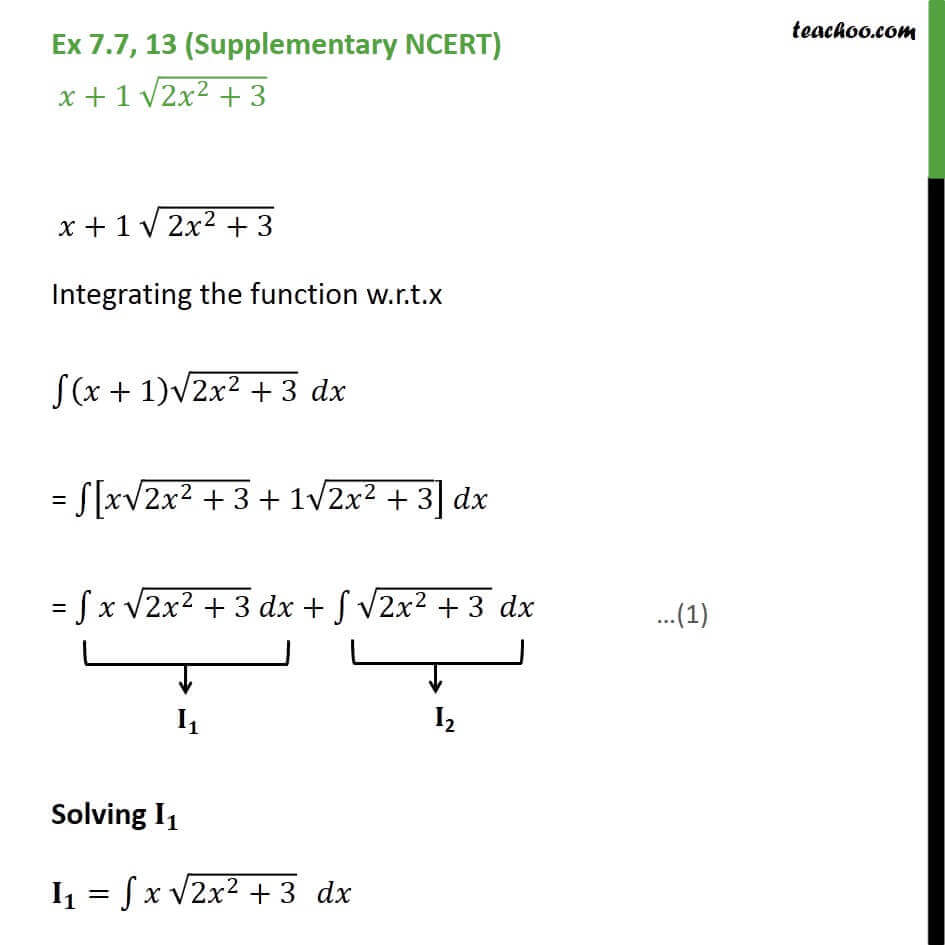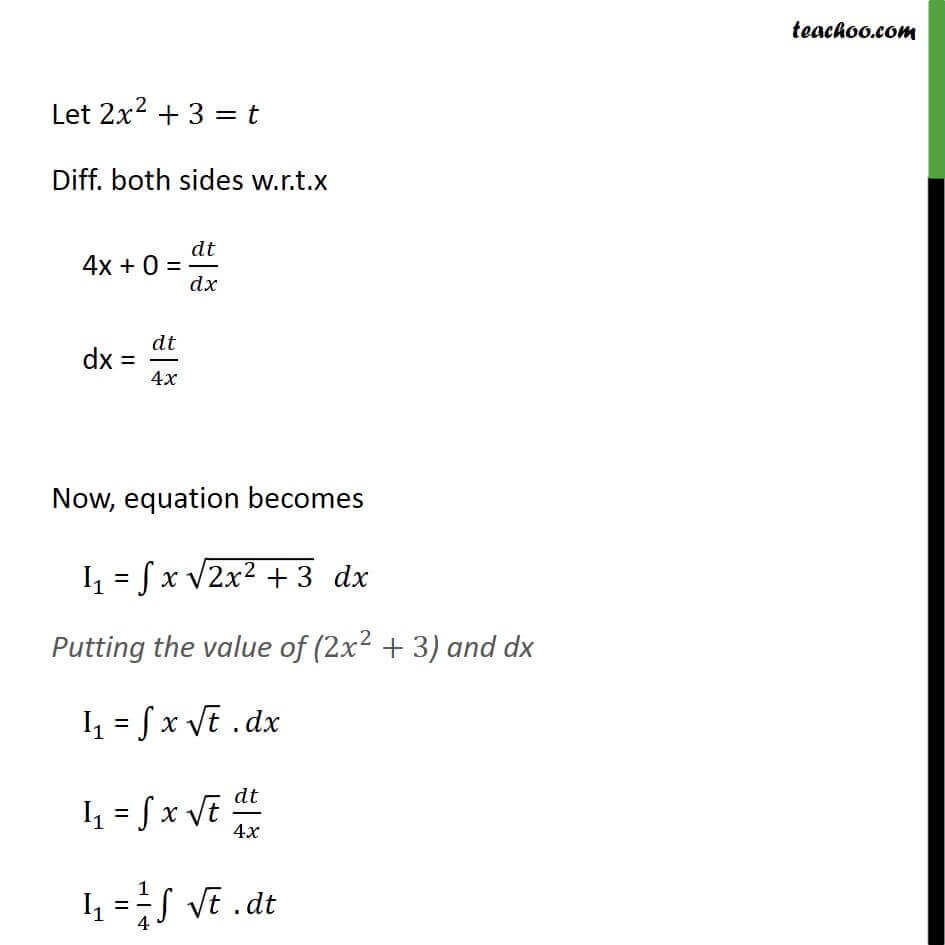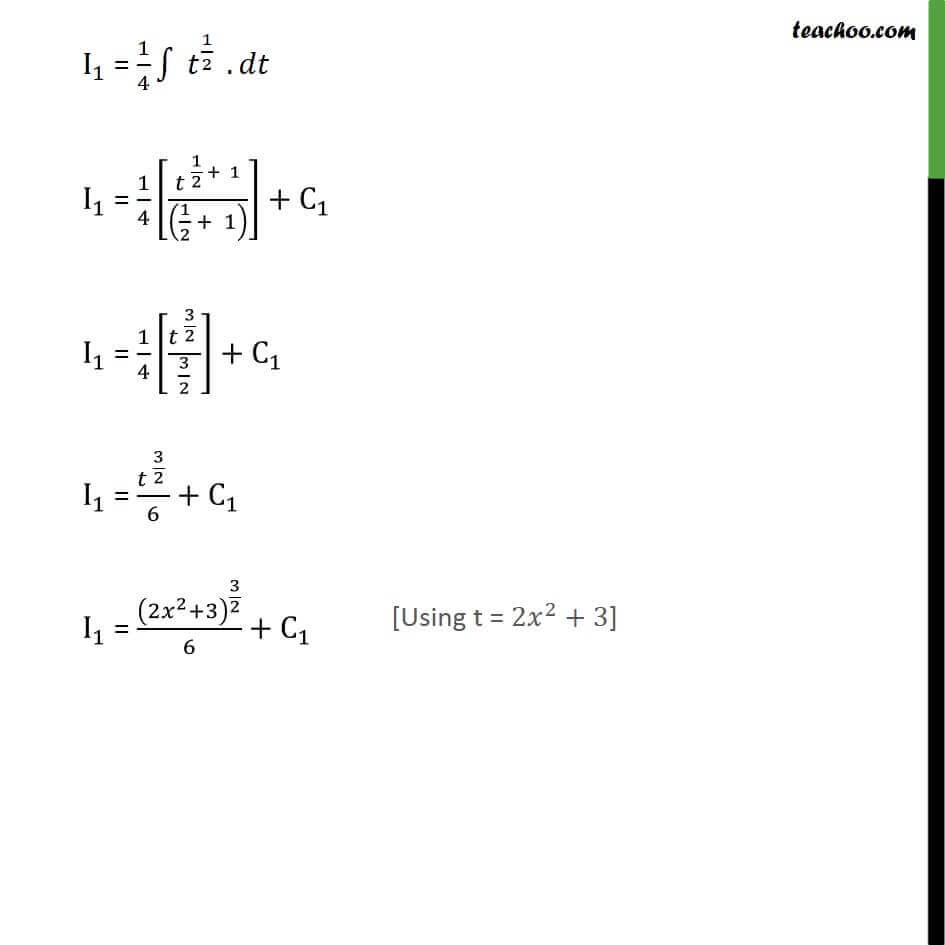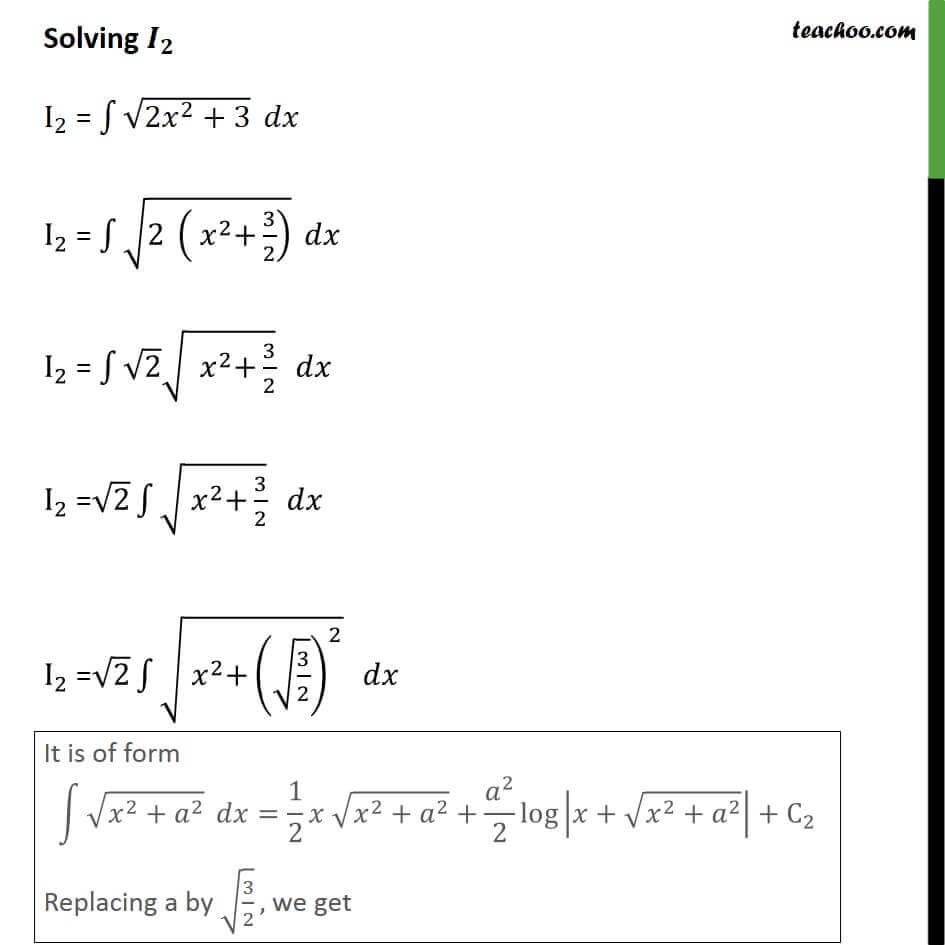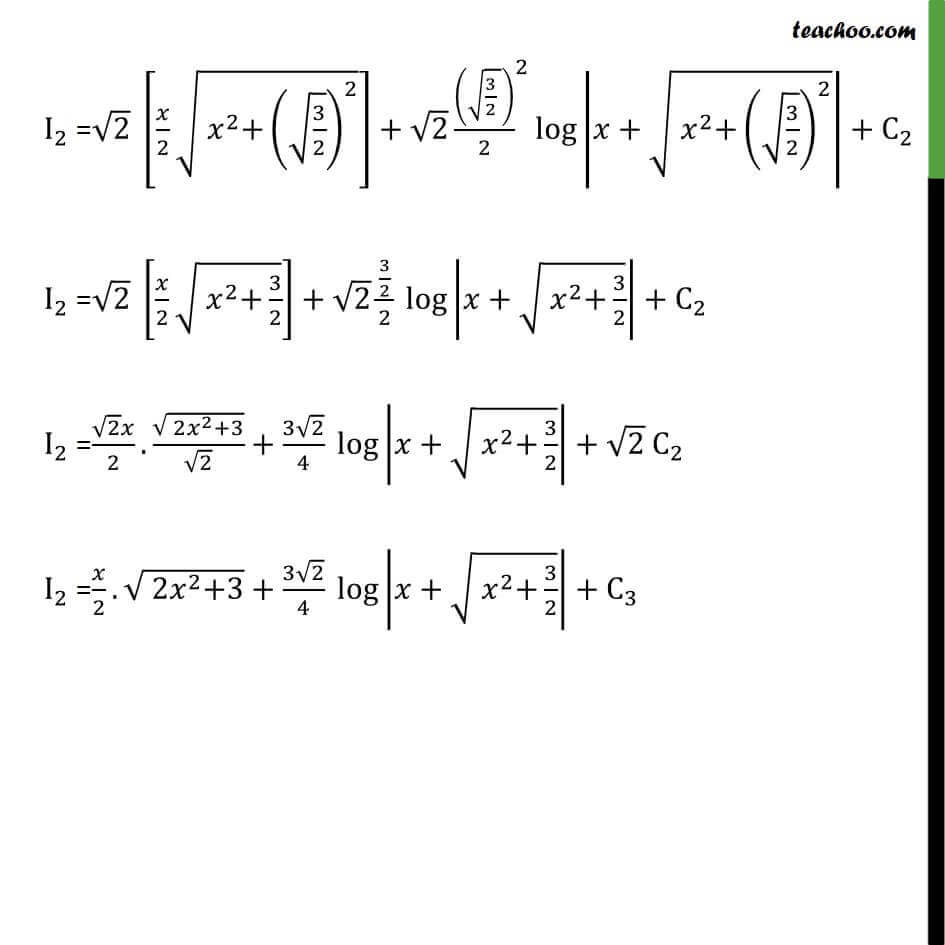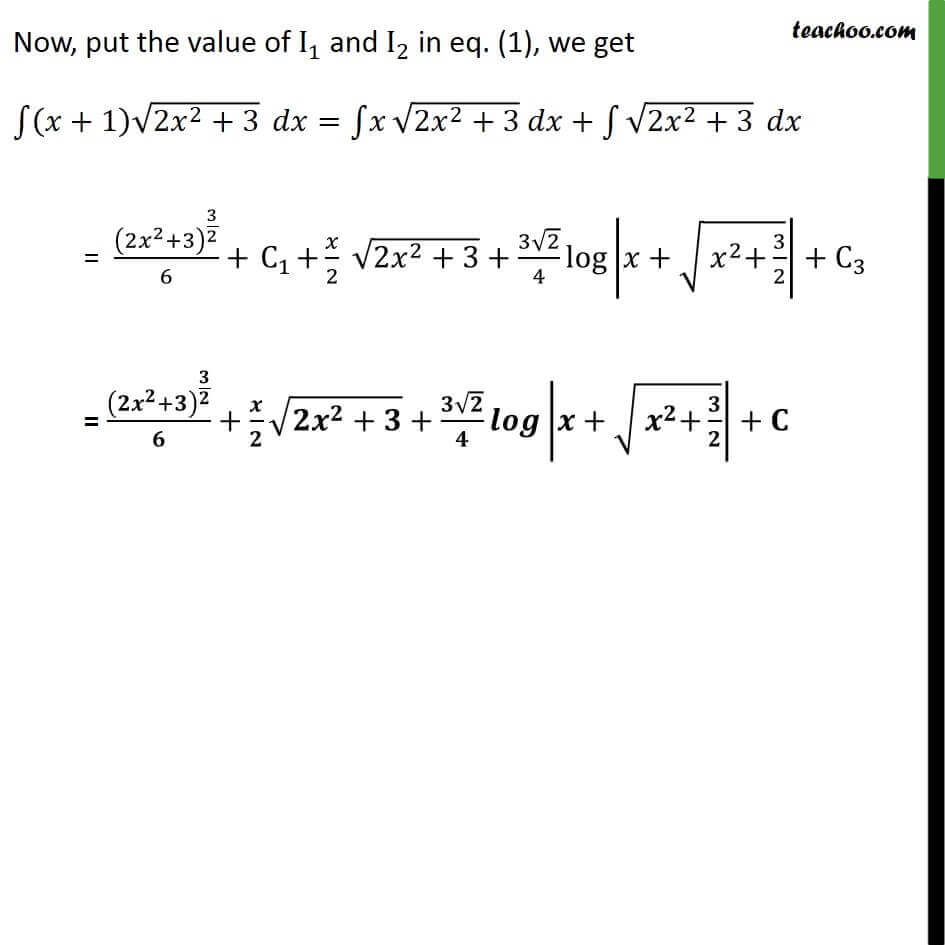Learn in your speed, with individual attention - Teachoo Maths 1-on-1 Class

### Transcript

Ex 7.7, 13 (Supplementary NCERT) 𝑥+1 √(2𝑥^2+3) 𝑥+1 √( 2𝑥^2+3) Integrating the function w.r.t.x ∫1▒〖(𝑥+1) √(〖2𝑥〗^2+3)〗 𝑑𝑥 = ∫1▒〖[𝑥√(〖2𝑥〗^2+3)+1√(〖2𝑥〗^2+3)] 𝑑𝑥〗 = ∫1▒〖𝑥 √(〖2𝑥〗^2+3) 𝑑𝑥+∫1▒〖√(〖2𝑥〗^2+3 ) 𝑑𝑥〗〗 Solving 𝐈_𝟏 𝐈_𝟏=∫1▒〖𝑥 √(〖2𝑥〗^2+3)〗 𝑑𝑥 Let 〖2𝑥〗^2+3=𝑡 Diff. both sides w.r.t.x 4x + 0 = 𝑑𝑡/𝑑𝑥 dx = 𝑑𝑡/4𝑥 Now, equation becomes I_1 = ∫1▒〖𝑥 √(〖2𝑥〗^2+3)〗 𝑑𝑥 Putting the value of (〖2𝑥〗^2+3) and dx I_1 = ∫1▒〖𝑥 √𝑡〗 . 𝑑𝑥 I_1 = ∫1▒〖𝑥 √𝑡〗 𝑑𝑡/4𝑥 I_1 = 1/4 ∫1▒〖 √𝑡 〗. 𝑑𝑡 I_1 = 1/4 ∫1▒〖 𝑡^(1/2) 〗 . 𝑑𝑡 I_1 = 1/4 [〖𝑡 〗^(1/2 + 1)/((1/2 + 1) )]+C_1 I_1 = 1/4 [〖𝑡 〗^(3/2 )/(3/2)]+C_1 I_1 = 〖𝑡 〗^(3/2 )/6+C_1 I_1 = (〖2𝑥〗^2+3)^(3/2)/6+C_1 Solving 𝑰_𝟐 I_2 = ∫1▒√(〖2𝑥〗^2+3) 𝑑𝑥 I_2 = ∫1▒√(2 (〖 𝑥〗^2+3/2) ) 𝑑𝑥 I_2 = ∫1▒〖√2 √( 〖 𝑥〗^2+3/2)〗 𝑑𝑥 I_2 =√2 ∫1▒√(〖 𝑥〗^2+3/2) 𝑑𝑥 I_2 =√2 ∫1▒√(〖 𝑥〗^2+(√(3/2))^2 ) 𝑑𝑥 It is of form ∫1▒〖√(𝑥^2+𝑎^2 ) 〗 𝑑𝑥=1/2 𝑥 √(𝑥^2+𝑎^2 )+𝑎^2/2 log⁡|𝑥+√(𝑥^2+𝑎^2 )|+C_2 Replacing a by √(3/2), we get I_2 =√2 [𝑥/2 √(〖 𝑥〗^2+(√(3/2))^2 )]+√2 〖((√(3/2 )))/2〗^2 log⁡|𝑥+√(〖 𝑥〗^2+(√(3/2))^2 )|+C_2 I_2 =√2 [𝑥/2 √(〖 𝑥〗^2+3/2)]+√2 (3/2)/2 log⁡|𝑥+√(〖 𝑥〗^2+3/2)|+C_2 I_2 =(√2 𝑥)/2.√(〖 2𝑥〗^2+3)/√2+(3√2)/4 log⁡|𝑥+√(〖 𝑥〗^2+3/2)|+〖√2 C〗_2 I_2 =𝑥/2.√(〖 2𝑥〗^2+3)+(3√2)/4 log⁡|𝑥+√(〖 𝑥〗^2+3/2)|+〖 C〗_3 Now, put the value of I_1 and I_2 in eq. (1), we get ∫1▒〖(𝑥+1) √(〖2𝑥〗^2+3)〗 𝑑𝑥=∫1▒𝑥 √(〖2𝑥〗^2+3) 𝑑𝑥+∫1▒√(〖2𝑥〗^2+3) 𝑑𝑥 = (〖2𝑥〗^2+3)^(3/2)/6+〖 C〗_1+𝑥/2 √(〖2𝑥〗^2+3)+(3√2)/4 log⁡|𝑥+√(〖 𝑥〗^2+3/2)|+〖 C〗_3 = (〖𝟐𝒙〗^𝟐+𝟑)^(𝟑/𝟐)/𝟔+𝒙/𝟐 √(〖𝟐𝒙〗^𝟐+𝟑)+(𝟑√𝟐)/𝟒 𝒍𝒐𝒈⁡|𝒙+√(〖 𝒙〗^𝟐+𝟑/𝟐)|+𝐂